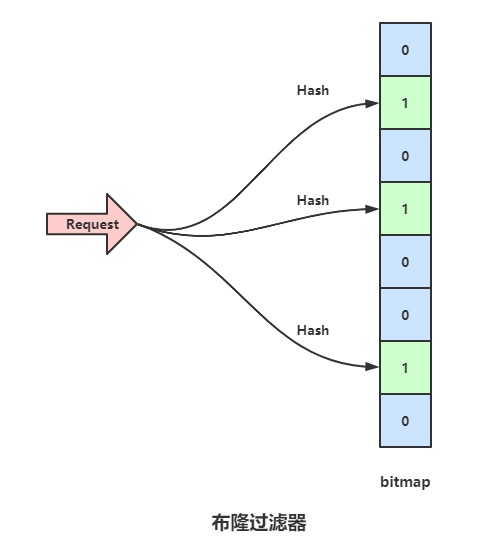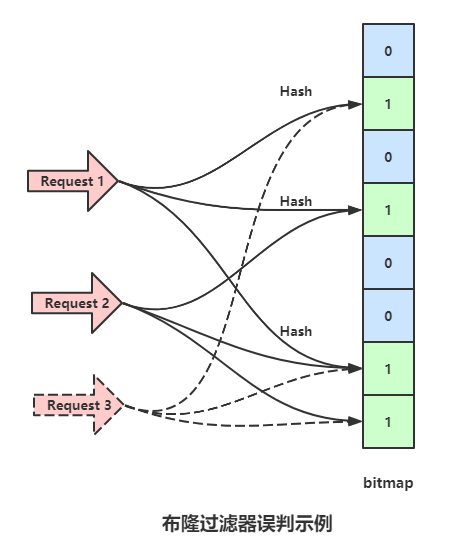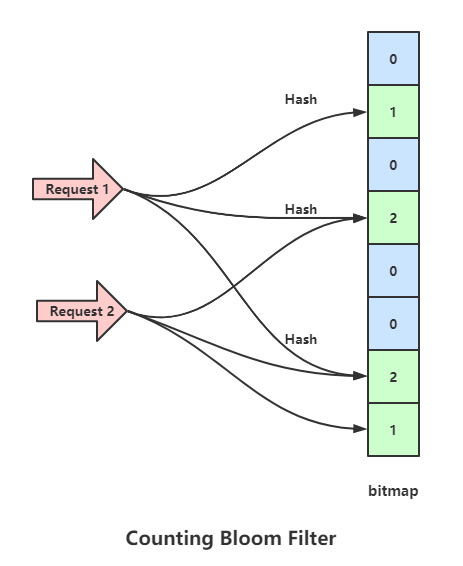## Redis布隆过滤器与布谷鸟过滤器

|     |   0 浏览
``````作者: 等不到的口琴

``````

# 布隆过滤器# 布隆过滤器的问题# 布隆过滤器增强版# 布谷鸟哈希

``````p1 = hash1(x) % l
p2 = hash2(x) % l
``````

# 布谷鸟过滤器

``````fp = fingerprint(x)
p1 = hash1(x) % l
p2 = hash2(x) % l
``````

``````fp = fingerprint(x)
p1 = hash(x)
p2 = p1 ^ hash(fp)  // 异或
``````

``````p1 = p2 ^ hash(fp)
``````

• 网站转载须在文章起始位置标注作者及原文连接，否则保留追究法律责任的权利。
• 公众号转载请联系网站首页的微信号申请白名单！